# We Helped With This Python Programming Assignment: Have A Similar One?

SOLVEDCategory Programming Python Undergraduate Solved Python Coding Help

## Short Assignment Requirements

I have an assignment on python. Please tell me if you are able to take it? Thanks.

## Assignment Description

Programming Assignment 2

In this assignment, you will solve a version of Sudoku using you own SAT solver.

What to submit: You should submit a single zip file that contains the following:Your program

(Optional) the contest folder

Part 0 DIMACS CNF file format.

This format is used to define a Boolean expression, written in conjunctive normal form (CNF), that may be used as an example of the satisfiability problem.

The satisfiability problem considers the case in which N boolean variables are used to form a Boolean expression involving negation (NOT), conjunction (AND) and disjunction (OR). The problem is to determine whether there is any assignment of values to the Boolean variables which makes the formula true. It's something like trying to flip a bunch of switches to find the setting that makes a light bulb turn on.

For simplicity, it is common to require that the boolean expression be written in conjunction normal form or "CNF". A formula in conjunctive normal form consists:

*  clauses joined by AND;

*  each clause, in turn, consists of literals joined by OR;

*  each literal is either the name of a variable (a positive literal,       or the name of a variable preceded by NOT (a negative literal.)

An example of a boolean expression in 3 variables and 2 clauses:

( x(1) OR ( NOT x(3) ) )

AND

( x(2) OR x(3) OR ( NOT x(1) ) ).

The CNF file format is an ASCII file format.

The file may begin with comment lines. The first character of each comment line must be a lower case letter "c". Comment lines typically occur in one section at the beginning of the file but are allowed to appear throughout the file.

The comment lines are followed by the "problem" line. This begins with a lower case "p" followed by a space, followed by the problem type, which for CNF files is "cnf", followed by the number of variables followed by the number of clauses.

The remainder of the file contains lines defining the clauses, one by one.

A clause is defined by listing the index of each positive literal, and the negative index of each negative literal. Indices are 1-based, and for obvious reasons, the index 0 is not allowed.

The definition of a clause may extend beyond a single line of text.

The definition of a clause is terminated by a final value of 0.

The file terminates after the last clause is defined.

Some of the examples of DIMACS CNF file can be found from SATLIB at UBC https://www.cs.ubc.ca/~hoos/SATLIB/benchm.html (https://www.cs.ubc.ca/%7Ehoos/SATLIB/benchm.html)

Example:

Here is the CNF file that corresponds to the simple formula discussed above:

c  simple_v3_c2.cnf      c      p cnf 3 2      1 -3 0

2 3 -1 0

### Part 1 Encode Sudoku game in CNF (Dimacs format)

#### The Sudoku game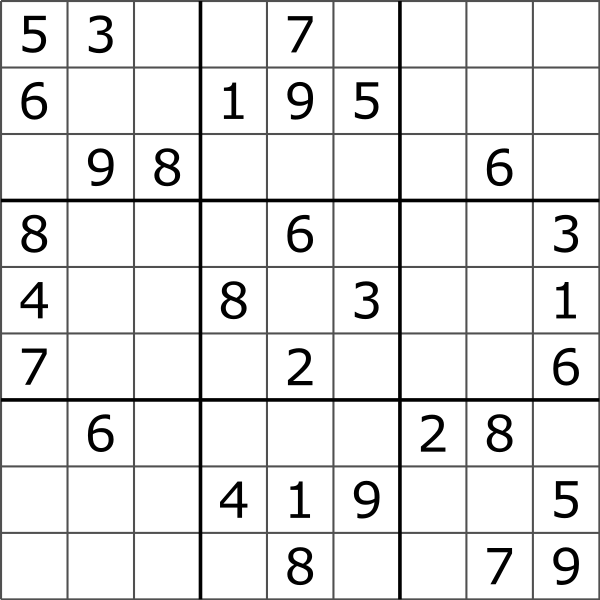For this assignment, we are solving a simplified sudoku without the box constraints. The simpler version of Sudoku is played on an NxN board (of course, N>0). The goal of the game is to place N copies of numbers 1 thru N on this board satisfying the following constraints in conjunction with constraints stemming from numbers already placed on the board, e.g., cell (1,1) contains 5.

1.  Each cell contains exactly one copy of any number.

2.  Each row contains every number exactly once,

i.e., there are no duplicate copies of a number in a row.    3. Each column contains every number exactly once,

i.e., there are no duplicate copies of a number in a column.

A solution to a given board will say which copy of a number should be placed in which cell.

#### Encoding

We will use one variable Vx,y,n to represent the presence of number n in cell (x, y). Since we have NxN number of cells that can contain any of the N unique numbers, we will have NxNxN number of variables, i.e., Vx,y,n with x, y, and n ranging from 1 thru N.

We can rewrite the above constraints as follows.

Each cell contains at least one copy of any number.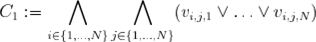Each cell contains at most one copy of any number.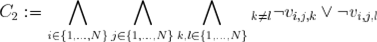No two fields in any column contain the same value.No two fields in any row contain the same valueNow we just need to include constraints that restrict cells that were labeled in the puzzle grid to keep that label. Let

S:={(i,j,k):cell (i,j) has label k in puzzle grid}.

Then the formula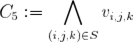ensures that the solution matches the original puzzle.

#### Putting it all together

So given a puzzle the formula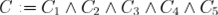is satisfied if and only if the variables vi,j,k describe a solution to the puzzle. Cell (i,j) is labeled k if and only if vi,j,k is true.

#### Q1(4 points)

Your job is to write a Python program that constructs the CNF formula C in Dimacs format from a sudoku grid.

Complete the empty function toCNF in sudoku.py. The function toCNF takes three arguments: the number N, an instance of sudoku of size NxN, and a string (for the name of output file).

For example, the instance for the picture above looks like this:

5  3 0 0 7 0 0 0 0

6  0 0 1 9 5 0 0 0

0 9 8 0 0 0 0 6 0

8 0 0 0 6 0 0 0 3

4 0 0 8 0 3 0 0 1

7 0 0 0 2 0 0 0 6

0 6 0 0 0 0 2 8 0

0 0 0 4 1 9 0 0 5

0 0 0 0 8 0 0 7 9

We use 0 to represent unoccupied cells.

After running the following commands

python sudoku.py -n 3 -i sudoku3_unsat.txt python sudoku.py -n 5

python sudoku.py -n 9 -i sudoku9.txt

Your code should quickly generate three files:

sudoku3_unsat.txt3.cnf

5.cnf sudoku9.txt9.cnf

Notice that it's ok to not specify an input instance, we will treat it as if we are inputting a file of all 0's.

We have included a binary executable of MiniSat solver for Linux. The MiniSat SAT solver is one of the fastest SAT solvers currently available.

You can check your encodings by:

./MiniSat_v1.14_linux sudoku3_unsat.txt3.cnf

./MiniSat_v1.14_linux 5.cnf

./MiniSat_v1.14_linux sudoku9.txt9.cnf

If your encoding is correct, only the first one is unsatisfiable. Feel free to do more testing with your own sudoku instances.

Every file generated by this command should be satisfiable:

python sudoku.py -n N

where N is any positive integer.

Remember You must include a line with a lower case "p" followed by a space, followed by "cnf", followed by the number of variables followed by the number of clauses before adding any CNF clauses.

### Part 2 DPLL SAT Solver

In this part, you will implement a basic DPLL SAT-solver in Python.

As a refresher—the motivation for a SAT solver is to determine if, for a given boolean formula, there exists an assignment of true and false to the variables such that the entire formula evaluates to true. Algorithms that solve the boolean satisfiability problem are employed by formal verification tools under-the-hood to determine the satisfiability of higher-level constraint models. In general, efficient SAT-solving is an extremely important and widely-used problem in computer science.

DPLL expects as input a formula in conjunctive normal form, i.e., a set of clauses that are joined together with “and”. This means that to satisfy the input clause set, every clause must be satisfied. We call a clause with only one literal a “unit clause” and a clause with no literals the “empty clause”, which is the same as falsehood. A literal is “pure” if, whenever it appears in the clause set, it always has the same sign. A set of unit clauses is consistent if no two have different signs for the same variable.

#### Input Specification

Your program should take in a formula in DIMACS CNF file format, where a formula is a list of clauses separated by 0s and each clause is a list of literals separated by spaces. Literals are non-zero numbers where a positive number denotes that the variable must be true and a negative number denotes it must be false. There is an implicit “or” between each literal and an implicit “and” between clauses.

In DPLLsat.py, we've provided the template for one possible implementation of reading input and internal clauses representation in python. Feel free to use it directly or implement your own.

#### Unit Propagation

The DPLL algorithm works by choosing an assignment of true or false for a variable, simplifying the formula based on that choice, then recursively checking the satisfiability of the simplified formula.

First, we’ll implement unit propagation, which is one aspect of this formula simplification. If a clause is a unit clause (one with only one variable), then the only way for the formula to be satisfiable is for that variable to be assigned consistently with its sign in that clause. Thus, we know that variable must take on that assignment, which tells us that all other clauses with that literal are now satisfied. In addition, we know that clauses with the literal’s negation will not be satisfied by that component, so we can remove the negation from all clauses.

The pseudocode for propagate-units is included below. First, write out a few test cases with your internal representation, and discuss them with a TA or another student. Then, implement and test this function.

propagate-units(F):

for each unit clause {+/-x} in F         remove all non-unit clauses containing +/-x         remove all instances of -/+x in every clause // flipped sign!

#### Pure Literal Elimination

The second aspect of formula simplification is eliminating pure literals (ones that occur with only one polarity throughout the formula). If a literal is pure, then each clause it appears in can be satisfied by assigning the variable consistent to the sign it appears with. Thus, without changing the satisfiability of the formula, we can add the pure literal as a unit clause and remove all other clauses is occurs in from the formula.

The pseudocode for pure-elim is included below. Again, write out some test cases and discuss them with a TA or another student. Then, implement and test this function.

pure-elim(F):

for each variable x         if +/-x is pure in F             remove all clauses containing +/-x             add a unit clause {+/-x}

#### Recursive Backtracking

After the DPLL employs these two simplification steps, it must pick some variable to branch on. The satisfiability problem is then split into two sub-problems: whether the formula is satisfiable with the chosen variable assigned as either true or false. Essentially, the algorithm “guesses” a variable to be true, recursively determines if that subproblem is satisfiable; if it is not, the algorithm then “guesses” the variable to be false and tries again.

You’re free to choose any reasonable method of picking variables to branch on. You might randomly select a variable, pick the variable that occurs the most in the formula, or even just take the alphabetically-next variable. By “reasonable” we mean a method that leads to correct results.

Note that if you do not include “involves all VARS” in the check below, DPLL will return a partial instance that potentially omits values for some variables that were removed in simplification steps. Since we’re changing the formula recursively, we need to remember the original set of variables (VARS).

solve(VARS, F):

F := propagate-units(F)     F := pure-elim(F)     if F contains the empty clause, return the empty clause // call this "unsat" in output     if F is a consistent set of unit clauses that involves all VARS, return F     x := pick-a-variable(F) // do anything reasonable here     if solve(VARS, F + {x}) isn't the empty clause, return solve(F + {x}) // works to have +x     else return solve(VARS, F + {-x}) // check -x

#### Q2 (6 points)

Implement the DPLL algorithm in DPLLsat.py.

You should be able to run your solver by:

python DPLLsat.py -i <inputCNFfile>

or

python DPLLsat.py -i <inputCNFfile> -v

if you want to see the solution.

OUTPUT specification:

Your program should print “UNSAT” if a formula is unsatisfiable and "SAT" if it is satisfiable.

Additionally, if verbosity is set to Ture, and the formula is satisfiable, the program should also print the solution in the form:

list of true literals list of false literals

where each literal is separated by a space and there is a newline between lists.

Verify your solver with the Minisat. Both solvers should output the same satisibility result for the same cnf instance.

#### (Optional) Sudoku solving contest (bonus 2 points)

The course contest involves solving the largest sudoku instance you can in 5 minutes.

##### Getting Started

You have 5 minutes to

1.  Generate a sudoku instance by:

python sudoku.py -n N

2.  Use your SAT solver to solve the problem:

python DPLLsat.py -i N.cnf

where N is a positive number.

##### What to submit

Put your modified sat solver and/or modified sudoku encoder in the contest folder.

Also, include a result.txt that reports the largest N you can solve in 5 minutes. You will also need to provide a brief description of what you did and include relevant references.

##### Restrictions

You are free to modify the DPLL solver. You can also work on improving the encoding of the Sudoku game. It's ok to implement advanced DPLL algorithms you found from internet/academic papers, but you need to provide the reference to them and write every line of the code by yourself!

WARNING: SAT solver optimization is a very time-consuming job. Please do so only if you have the interest and time!

While we won't validate your result, we still expect you not to falsely represent your work. Please don't let us down.

### Part 3 Feedback

Give one short piece of feedback about the course so far. What have you found most interesting? Is there a topic that you had trouble understanding? Are there any changes that could improve the value of the course to you?

How many hours did you spend on this assignment?

*Instruction for DPLL SAT solver is adopted from the Logic for Systems course offered at Brown University.

Is it free to get my assignment evaluated?

Yes. No hidden fees. You pay for the solution only, and all the explanations about how to run it are included in the price. It takes up to 24 hours to get a quote from an expert. In some cases, we can help you faster if an expert is available, but you should always order in advance to avoid the risks. You can place a new order here.

How much does it cost?

The cost depends on many factors: how far away the deadline is, how hard/big the task is, if it is code only or a report, etc. We try to give rough estimates here, but it is just for orientation (in USD):

 Regular homework \$20 - \$150 Advanced homework \$100 - \$300 Group project or a report \$200 - \$500 Mid-term or final project \$200 - \$800 Live exam help \$100 - \$300 Full thesis \$1000 - \$3000

How do I pay?

Credit card or PayPal. You don't need to create/have a Payal account in order to pay by a credit card. Paypal offers you "buyer's protection" in case of any issues.

Why do I need to pay in advance?

We have no way to request money after we send you the solution. PayPal works as a middleman, which protects you in case of any disputes, so you should feel safe paying using PayPal.

Do you do essays?

No, unless it is a data analysis essay or report. This is because essays are very personal and it is easy to see when they are written by another person. This is not the case with math and programming.

Why there are no discounts?

It is because we don't want to lie - in such services no discount can be set in advance because we set the price knowing that there is a discount. For example, if we wanted to ask for \$100, we could tell that the price is \$200 and because you are special, we can do a 50% discount. It is the way all scam websites operate. We set honest prices instead, so there is no need for fake discounts.

Do you do live tutoring?

No, it is simply not how we operate. How often do you meet a great programmer who is also a great speaker? Rarely. It is why we encourage our experts to write down explanations instead of having a live call. It is often enough to get you started - analyzing and running the solutions is a big part of learning.

What happens if I am not satisfied with the solution?

Another expert will review the task, and if your claim is reasonable - we refund the payment and often block the freelancer from our platform. Because we are so harsh with our experts - the ones working with us are very trustworthy to deliver high-quality assignment solutions on time.

Customer Feedback

"Thanks for explanations after the assignment was already completed... Emily is such a nice tutor! "

Order #13073